#Coffee Space

Listen:

## OS Compression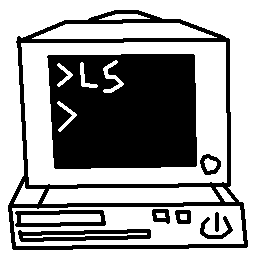Preview Image

### Introduction

Recently, I found myself wondering about writing a smaller, bare-bones operating system for the pure challenge of the task. Writing bloat software is one thing, but to write a lean thing of beauty is quite another.

There are several attractions to writing a small OS:

• There is a point when you can say "it's done enough"
• It's a relatively small project, so not too time consuming
• Looks awesome once it's done

I am also looking into writing a book on the subject, something that people can interact with and should be a relatively small project. I feel as if most people want the experience, but don't want to be engulfed by the art of writing an OS. I think writing a small, self-contained OS is ideal.

I have already written the Sax Operating System, with a bootloader/kernel bootloader in 512 bytes and have have come against, what I considered at the time, to be a pretty hard limit of how many features can be supported is such a small space.

This was until an idea occurred, which is where we are going with this article.

### Analysis

As any good scientist would, we need to analyse the data we have. We're going to look at compressing some data we already have, in this case files from the older operating system mentioned previously. We're going to be using the following files:

These have been chosen as popular features from the old OS, hopefully being representative of the code we wish to write for the new OS.

The following Java code is used to analyse the binary data:

``````import java.io.File;
import java.io.FileInputStream;
import java.io.IOException;

/**
* Main.java
*
* This class represents the entire program, responsible for quickly analysing
* binary blob data.
**/
public class Main{
private static final String help =
"\n[OPT] [FILE]..[FILE]" +
"\n" +
"\n  OPTions" +
"\n" +
"\n    -h --help       Display this help" +
"\n    -v --version    Display program version" +
"\n" +
"\n  FILE" +
"\n" +
"\n    The binary file/s to be checked.";
private static final String version = "v0.0.1";

/**
* main()
*
* Initialise the main program here and parse arguments to the Main object.
*
* @param args The arguments to the program.
**/
public static void main(String[] args){
new Main(args);
}

/**
* Main()
*
* Responsible for processing the command line arguments and processing, if
* required.
*
* @param args The arguments to the program.
**/
public Main(String[] args){
/* Initialise variables */
String[] files = null;
int size = 512;
/* Process command line arguments */
for(int x = 0; x < args.length; x++){
switch(args[x]){
case "-h" :
case "--help" :
exit(help);
break;
case "-s" :
case "--size" :
try{
size = Integer.parseInt(args[++x]);
}catch(NumberFormatException e){
exit(help);
}
break;
case "-v" :
case "--version" :
exit(version);
break;
default :
if(files == null){
files = new String[args.length - x];
}
files[args.length - x - 1] = args[x];
break;
}
}
/* Make sure there is a file to process */
if(files != null){
/* Create the buffer */
byte[] buffer = new byte[size * files.length];
/* Iterate over the files */
for(int x = 0; x < files.length; x++){
File f = new File(files[x]);
/* Make sure the file exists */
if(!f.isFile()){
exit(help);
}
try{
FileInputStream fis = new FileInputStream(f);
fis.close();
}catch(IOException e){
exit(help);
}
}
/* Analyse */
System.out.println("# analyse1Pairs #");
analyseNPairs(buffer, 1);
System.out.println("# analyse2Pairs #");
analyseNPairs(buffer, 2);
System.out.println("# analyse3Pairs #");
analyseNPairs(buffer, 3);
System.out.println("# duplicate2Pairs #");
duplicateNPairs(buffer, 2);
System.out.println("# duplicate3Pairs #");
duplicateNPairs(buffer, 3);
System.out.println("# duplicate4Pairs #");
duplicateNPairs(buffer, 4);
System.out.println("# nSpacing #");
nSpacing(buffer);
}else{
exit(help);
}
}

/**
* analyseNPairs()
*
* Analyse N pairs of bytes in the data stream.
*
* @param data The data to be checked.
* @param n The number of pairs to be checked, where N is less than or equal
* to three.
**/
private void analyseNPairs(byte[] data, int n){
/* Initialise the frequency array */
int[] freq = new int[(int)(Math.pow(256, n))];
for(int x = 0; x < freq.length; x++){
freq[x] = 0;
}
/* Iterate over the pairs, increase the frequency */
for(int x = 0; x < data.length - n + 1; x++){
/* Calculate the index value */
int index = 0;
for(int y = 0; y < n; y++){
int t = (int)(data[x + (n - y - 1)]);
index += Math.pow(256, y) * (t < 0 ? 127 - t : t);
}
/* Increase the frequency */
++freq[index];
}
/* Output the results */
for(int x = 0; x < freq.length; x++){
/* NOTE: Only print if a result greater than zero. */
if(freq[x] > 0){
System.out.println(x + "\t" + freq[x]);
}
}
}

/**
* duplicateNPairs()
*
* Analyse N duplicate pairs of bytes in the data stream.
*
* @param data The data to be checked.
* @param n The number of duplicate pairs to be checked.
**/
private void duplicateNPairs(byte[] data, int n){
/* Initialise the frequency array */
int[] freq = new int;
for(int x = 0; x < freq.length; x++){
freq[x] = 0;
}
/* Iterate over the pairs, increase the frequency */
for(int x = 0; x < data.length - n + 1; x++){
/* Calculate the index value */
boolean equal = true;
for(int y = 1; y < n; y++){
equal &= data[x] == data[x + y];
}
/* Check whether values are equal */
if(equal){
/* Increase the frequency */
++freq[((int)data[x] < 0 ? 127 - (int)data[x] : (int)data[x])];
}
}
/* Output the results */
for(int x = 0; x < freq.length; x++){
/* NOTE: Only print if a result greater than zero. */
if(freq[x] > 0){
System.out.println(x + "\t" + freq[x]);
}
}
}

/**
* nSpacing()
*
* Analyse the frequency of which pairs can be found with N spacing.
*
* @param data The data to be checked.
**/
private void nSpacing(byte[] data){
int BYTE_SIZE = 256;
/* Initialise the frequency array */
int[][] freq = new int[BYTE_SIZE][];
for(int y = 0; y < freq.length; y++){
freq[y] = new int[BYTE_SIZE];
for(int x = 0; x < freq[y].length; x++){
freq[y][x] = 0;
}
}
/* Iterate over the pairs, increase the frequency */
for(int o = 0; o < data.length; o++){
/* Iterate over the spacing tests */
for(int n = 1; n < BYTE_SIZE && n + o < data.length; n++){
/* Check if current character is found after N spaces */
if(data[o] == data[o + n]){
++freq[n][((int)data[o] < 0 ? 127 - (int)data[o] : (int)data[o])];
}
}
}
/* Output the results */
for(int y = 0; y < freq.length; y++){
for(int x = 0; x < freq[y].length; x++){
/* Only print not zero results */
if(freq[y][x] != 0){
System.out.println(y + "\t" + x + "\t" + freq[y][x]);
}
}
}
}

/**
* exit()
*
* Hard exit the program with a message.
*
* @param msg The message to be displayed.
**/
private void exit(String msg){
System.out.println(msg);
System.exit(0);
}
}``````

NOTE: LibreOffice graphs can be found here.

#### 1 Byte Frequency

First things first, we need to find out how this binary data is distributed: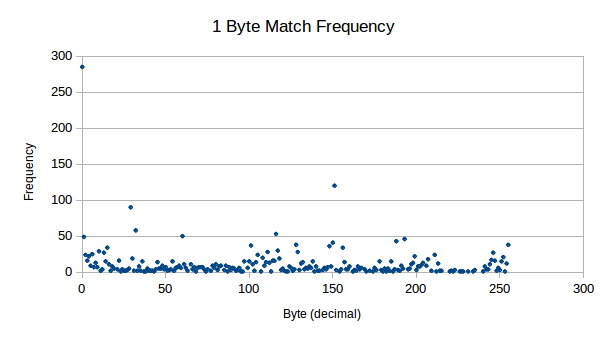1 byte frequency distribution

For only a little work, this is some nice results to see. Some interesting points for me:

• 285 counts out of 2,560 bytes (11.1% of bytes) for `0d`
• 31 unused bytes (frequency count of zero)
• Only 1 byte is >255 counts

From this, we can conclude that this is compressible data!

#### 2 and 3 Byte Frequency

Here we analyse the possibility of some dictionary related lookup system, by analysing how pairs of data is distributed in our sample sets. The result is stored as a decimal, where we only keep frequencies larger than zero. This is because when originally testing the software, for some reason the Java heap doesn't like 256 ^ 4 lookup tables, not sure why (sarcasm).2 byte frequency distribution

As you can see from these results, it should certainly be possible for compression on 2 byte pairs in our data, even from small 512 byte chunks.3 byte frequency distribution

Next, we see 3 byte pairs, which also show decent levels of frequency. These don't seem to tend much higher than "background noise" for all aspects other than `0`.

In order for a dictionary method of compression to work, we can first make some assumptions about in order to theoretically test feasibility:

• The implementation of decompression is 50 bytes in size (a number from thin air).
• We do 3 byte matching, replacing each 3 bytes with one unused place-holder byte.
• Description of replacement requires 1 unused byte + 3 byte drop in.

We can now calculate how much data we need to replace in order to make the process worth our time:

• Overhead, c = -50 bytes
• Compression, m = 3 bytes
• Bytes saved, y = ?
• Matches, x = ?

This can now be expressed as `y = mx + c`, or `y = (3 * x) - 50`. In order to break even, so that `y = 0`, we must calculate `50 / 3 = x`, which is 17 matches. In order to save 50 bytes, comparable to the implementation of the decompression algorithm, we must calculate where `y = 50`, so that `(50 + 50) / 3`, which is 34 matches. In just 512 bytes, this is a push.

Of course, this doesn't take into consideration the data required to describe the replacements, further eating into any gains we may have. In order for this system to work, there must be a high amount of overlapping dictionary mappings, otherwise the description of the replacement will cost more than the gain from the replacement.

#### 2 Byte Duplicates

Yet another interesting pattern (a subset pattern from the one discussed previously) in the data is where we see duplicates of data side-by-side with the same value. Consider the following results: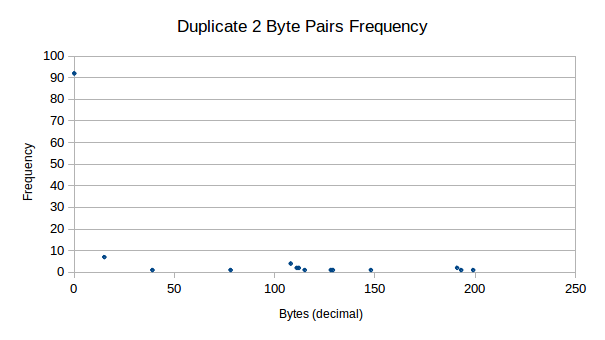2 byte duplicate frequency

We see that firstly, we have many duplicate zeros in our data. Other than this, there are still very strong presences of duplicate data for other values, mainly for `15d`, where we see 7 strong counts.

There are some benefits to duplicate representation:

• Description count is 2 bytes, not 3 bytes as you can assume you know the second byte
• Easier to find duplicate data and will always occur with a given probability

On the negative side, duplicates are much less likely to occur in 125 bytes. Somewhere between `46 / 285` and `92 / 285` of our data is side-by-side zeros, representing space that we can fit more programming code in anyway. This greatly reduces the benefit of using this method.

#### 3 and 4 Byte Duplicates

These tables are now too small to even produce a graph that has any advantage, so we display our raw results here:

``````Duplicate Match_Index Frequency
3         0           72
3         15          6
4         0           65
4         15          5``````

Specifically, byte `15d` is of interest, as this byte seems highly compressible. Assuming this value is related to an opcode, `0x0F` seems to be used for popping data from the stack Wikipedia. This would mean that in our code, after using one `pop` instruction, we are quite likely to use another - which is certainly an interesting find.

#### N Spacing

Here we decide to look at characters that repeat after N spaces, the idea being that we can compress two bytes down into one byte that describes the current character and it's repeat location. A example of this is below:

``````X  = 'a'  2
S  = 'a' 'b' 'c' 'a' 'd'
S' =  X  'b' 'c' 'd'``````

So in this example, the description is that `X` denotes that the character `'a'` exists in the place of `X`, but also in an offset of `2` characters. Used in the example string `S`, we see that we are able to compress our string to `S'`. In out testing, we limit the spacing to 255 spaces, as this still easily fits in an 8 bit byte and makes the data description smaller to work with.

Now we analyse the results: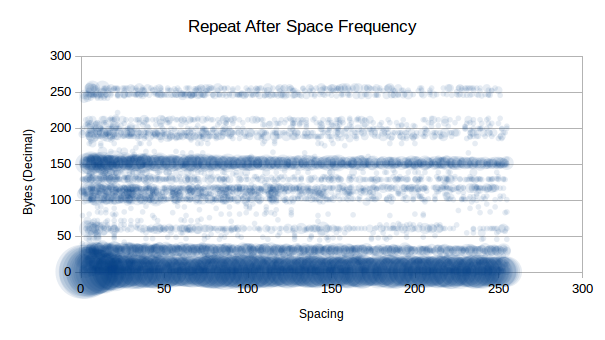N spacing frequency

As you can see, `0d` is clearly highly compressible as we saw in previous tests, but now we see that there are some other highly compressible data that don't repeat immediately, such as `150d`. Interestingly that is not an x86 instruction.

A list interesting (repeats 5 or more times) repeats include:

• `0d` - No spacing
• `1d` - Possible VM call
• `10d` - New line
• `13d` - Carriage return
• `15d` - Pop instruction
• `29d` - No information
• `32d` - Space character or logical AND instruction
• `60d` - No information
• `101d` - No information
• `116d` - No information
• `117d` - No information
• `150d` - No information
• `151d` - No information
• `156d` - Push flags instruction
• `188d` - No information
• `251d` - Set interrupt flag instruction
• `255d` - Call or decrement or increment or jump instruction

Looking past the highly compressible `0d`, which will be unlikely to be in our final compression data, we the following order of compressible data (just some):

• `151d` at `20` spaces is compressible `20` times
• `150d` at `6` spaces is compressible `16` times
• `29d` at `13` spaces is compressible `11` times
• `60d` at `4` spaces is compressible `9` times
• `255d` at `7` spaces is compressible `7` times
• `117d` at `10` spaces is compressible `7` times
• `32d` at `7` spaces is compressible `7` times

We potentially have 31 bytes at our disposal to represent N-spaced repeats, so as long as the savings are greater than the cost of representation (`<INDICATOR>` + `<CHAR>` + `<SPACING>`), then we shall see savings.

Assuming we get half of the saving mentioned in our list, that would be:

``(20 + 16 + 11 + 9 + 7 + 7 + 7) / 2 = 38.5 ~= 38 bytes``

That 38 bytes saved, minus the representation bytes, `7 * 3 = 21`, leaves us a saving off 17 bytes. Of course, the implementation of the decompression also will have a cost, meaning we will use more bytes than we save with any reasonably sized OS. Back to the drawing board!

### Compression

#### Implementations

##### Bubble Sort Compression

So a crazy idea I had was to implement a bubble-sort inspired compression algorithm, something that seemed on paper that may have some merit. I decided to write a program to explore the idea, so that I could approach this idea with a Scientific approach.

Here is the test program:

``````import time

debug = False

# ordered()
#
# Check if the data is ordered.
#
# @param data The stream to be tested.
# @return True if ordered, otherwise False.
def ordered(data) :
for x in range(0, len(data) - 1) :
if data[x] > data[x + 1] :
return False
return True

# decompressed()
#
# Check whether the data has been decompressed.
#
# @param data The stream to be tested.
# @return True if decompressed, otherwise False.
def decompressed(data) :
for x in range(0, len(data) - 1) :
if data[x] == float("inf") :
return False
return True

# order()
#
# Orders a set of data. Functional method.
#
# @param data The data stream to be tested.
# @return The ordered data.
def comp(data) :
# Initialise variables
indx = 0
# Infinite loop until complete
while 1 :
# Display what we have
if debug == True :
print(str(indx) + "::" + str(data))
# Make sure we're not at the end
if ordered(data) == True :
return data
# Check if we're at the end
if indx >= len(data) - 1 :
indx = 0
# Check whether ordering required for this pair
if data[indx] > data[indx + 1] :
tmp = data[indx]
data[indx] = data[indx + 1]
data[indx + 1] = tmp
data.insert(indx + 2, float("inf"))
# Next check
indx += 1
# Should never reach here!
print("ERROR!")

# decomp()
#
# Decompress the data stream. Functional method.
#
# @param data The data stream to be tested.
# @return The unordered data.
def decomp(data) :
# Initialise variables
indx = 0
# Infinite loop until complete
while 1 :
# Display what we have
if debug == True :
print(str(indx) + "::" + str(data))
# Make sure we're not at the end
if decompressed(data) == True :
return data
# Check if we're at the end
if indx <= 2 :
indx = len(data) - 1
# Check whether ordering required for this pair
if data[indx] == float("inf") :
tmp = data[indx - 1]
data[indx - 1] = data[indx - 2]
data[indx - 2] = tmp
data.pop(indx)
# Next check
indx -= 1
# Should never reach here!
print("ERROR!")

tests = [
[4, 2, 1, 3],
[4, 3, 2, 1],
[3, 4, 1, 2],
[3, 2, 4, 1],
[1, 2, 1, 2],
[2, 1, 2, 1],
[4, 2, 1, 3, 4],
[4, 2, 1, 3, 4, 3],
[4, 2, 1, 3, 4, 3, 2],
[4, 2, 1, 3, 4, 3, 2, 1],
[4, 2, 1, 3, 4, 3, 2, 1, 3],
[4, 2, 1, 3, 4, 3, 2, 1, 3, 4],
[4, 2, 1, 3, 4, 3, 2, 1, 3, 4, 1],
[4, 2, 1, 3, 4, 3, 2, 1, 3, 4, 1, 2],
[4, 2, 1, 3, 4, 3, 2, 1, 3, 4, 1, 2, 3]
]

print("TEST,INITIAL_SIZE,START_TIME,DEFLATED_SIZE,COMPRESSION_TIME,DECOMPRESSION_TIME,RESULT")
for x in range(0, len(tests)) :
output = "\"" + str(tests[x]) + "\","
output += str(len(tests[x])) + ","
output += str(time.time()) + ","
c = comp(tests[x])
output += str(len(c)) + ","
output += str(time.time()) + ","
d = decomp(c)
output += str(time.time()) + ","
if c == d :
output += "EQUAL"
else :
output += "NOT_EQUAL"
# Output the results
print(output)``````

This produced the following results (original here):

``````SIZE DEFLATE COMPRESS        DECOMPRESS
4    15      0               0
4    21      0               0
4    13      0               0
4    15      0               0
4    6       0               0
4    11      0               0
5    27      0               0
6    53      0               0
7    116     0.0099999905    0
8    289     0.0700001717    0
9    574     0.6699998379    0
10   1140    4.9600000381    0.0099999905
11   2526    46.3900001049   0.0199999809
12   5105    522.2400000095  0.129999876
13   10206   5851.7400000095 0.7999999523``````

Now we can now take a look at the feasibility of the project and whether we are looking at a good compression method or if this is not feasible.Compression Size

Here we are looking at initial size vs deflated size, the purpose of this is to look at RAM usage from decompression. This doesn't initially look too bad, until you plug in `x = 512` (512 bytes to compress), giving you ~1x10^167... Oh my, this is a good start. We won't stop here, there may be some technique to compress this is RAM during decompression - so we shan't worry too much about this.Compression Time

This is a show stopper - it looks as if no current computer could even compress 512 bytes using this method, in some human feasible time. By the looks of this, it could literally take a lifetime to compute the compressed data set.Decompression Time

The decompression starts with small values, but appears as though it too would become an extremely time consuming process wants extended to 512 bytes worth. The following graph allows us to make a decision as to whether this will be an issue for us.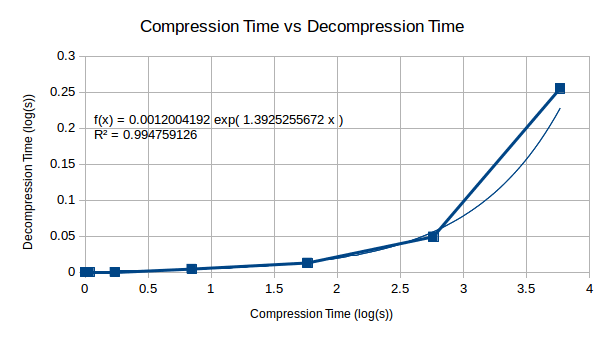Compression vs Decompression

Looking at the relationship between compression and decompression, we see that compression becomes a much harder problem than decompression. This suggests that although the compression complexity is high for this algorithm, decompression will become less complex in comparison and may be computable on a reasonably well powered machine.

To conclude, there are two major problems with this compression algorithm that make it useless until solved:

1. RAM requirements for algorithm are exceptionally high.
2. The compression of the data itself takes an exceptionally long time.

It looks as though, for now, this one is off the table until a later date. This is why we test ;)

### Current Progress

• `[X]` Analyse frequency of bytes
• `[X]` Analyse frequency of 2-pair repeat bytes
• `[X]` Analyse frequency of 3-pair repeat bytes
• `[X]` Analyse duplicate frequency of 2-pair repeat bytes
• `[X]` Analyse duplicate frequency of 3-pair repeat bytes
• `[X]` Analyse duplicate frequency of 4-pair repeat bytes
• `[X]` Analyse N spacing repeat frequency
• `[ ]` Research compression algorithms
• `[~]` Design compression algorithms